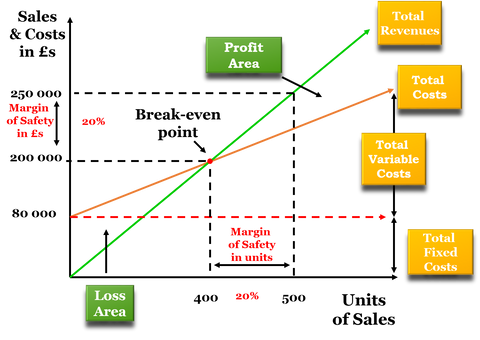Welcome to our website!

# Cost Volume Profit Analysis (CVP) / Break Even Analysis (Part 1)

## The Profit Equation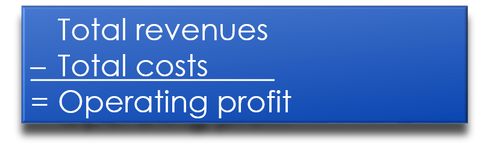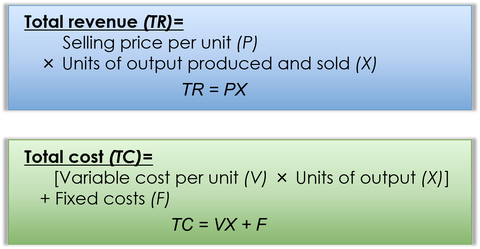## Contribution Margin

### Basically CM is the difference between price and variable cost. It is what is leftover to cover fixed costs and then add to operating profit.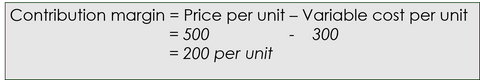## Contribution Margin Ratio

### The contribution margin ratio is the contribution margin as a percentage of sales revenue.## Break Even Analysis

### In summary, at break-even target profit is zero. Therefore, break-even sales volume in units equals fixed costs divided by unit contribution margin and break-even sales volume in dollars equals fixed costs divided by contribution margin ratio.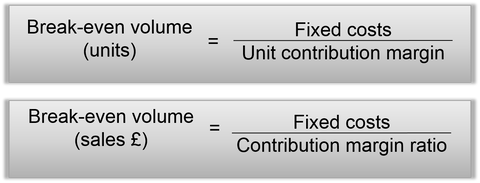## Target Profit Analysis

### In addition to calculating the break-even level of sales, a company can set itself a target to achieve a certain level of profits.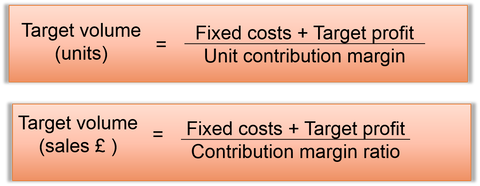## Margin of Safety

### Margin of safety as a percentage equals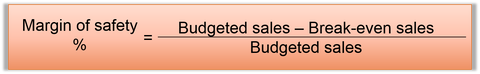## Break Even Chart

### The green line is Total Revenues for every unit sold, the red line is Total Cost for every unit sold. The point where these two lines intersect is the Break Even Point.